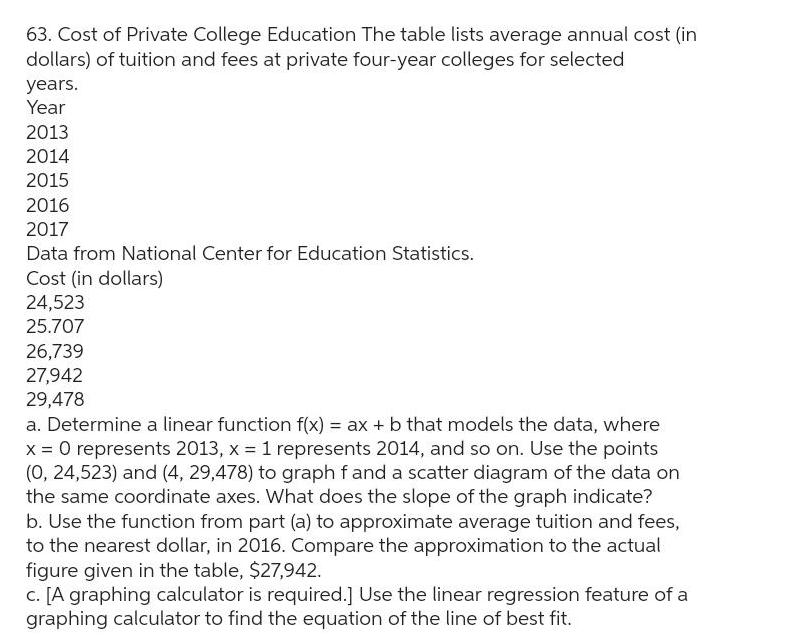Question:

# 63 Cost of Private College Education The table lists average

Last updated: 9/12/202363 Cost of Private College Education The table lists average annual cost in dollars of tuition and fees at private four year colleges for selected years Year 2013 2014 2015 2016 2017 Data from National Center for Education Statistics Cost in dollars 24 523 25 707 26 739 27 942 29 478 a Determine a linear function f x ax b that models the data where x 0 represents 2013 x 1 represents 2014 and so on Use the points 0 24 523 and 4 29 478 to graph f and a scatter diagram of the data on the same coordinate axes What does the slope of the graph indicate b Use the function from part a to approximate average tuition and fees to the nearest dollar in 2016 Compare the approximation to the actual figure given in the table 27 942 c A graphing calculator is required Use the linear regression feature of a graphing calculator to find the equation of the line of best fit